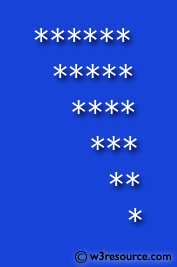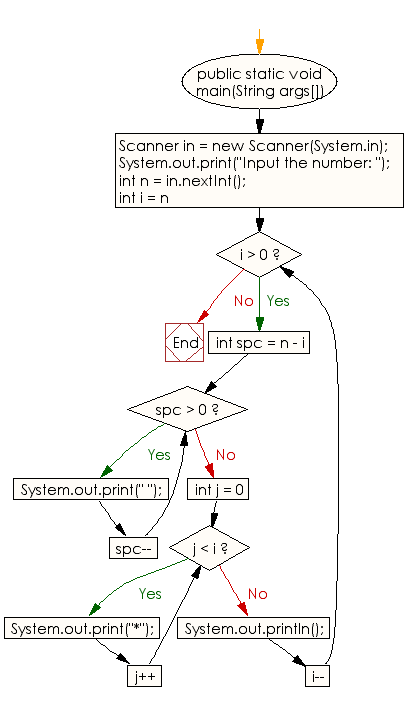﻿ Java exercises: Generate a following *'s triangle - w3resource# Java Conditional Statement Exercises: Generate a following *'s triangle

## Java Conditional Statement: Exercise-23 with Solution

Write a java program to generate a following *'s triangle.

Test Data
Input number of rows: 6

Pictorial Presentation:Sample Solution:

Java Code:

``````import java.util.Scanner;
public class Exercise23 {
public static void main(String args[])
{
Scanner in = new Scanner(System.in);
System.out.print("Input the number: ");
int n = in.nextInt();
for (int i = n; i > 0; i--)
{
for (int spc = n - i; spc > 0; spc--)
{
System.out.print(" ");
}
for (int j = 0; j < i; j++)
{
System.out.print("*");
}
System.out.println();
}
}

}
```
```

Sample Output:

```Input the number: 6
******
*****
****
***
**
*
```

Flowchart:Java Code Editor: